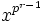# Cauchy's theorem

## Statement

If$G$ is a finite group, and$p$ is a prime number dividing the order of$G$, then$G$ has a subgroup of order exactly$p$. In particular,$G$ has an element of order exactly$p$.

## Facts used

1. Sylow subgroups exist: For any finite group$G$ and any prime$p$,$G$ has a subgroup whose order is the largest power of$p$ dividing$G$. Such a subgroup is termed a$p$-Sylow subgroup.

## Proof

### Proof using existence of Sylow subgroups

Given: A finite group$G$, a prime divisor$p$ of the order of$G$.

To prove:$G$ has a subgroup of order exactly$p$.

Proof: By fact (1),$G$ has a$p$-Sylow subgroup, say$P$. Since$p$ divides the order of$G$,$P$ is nontrivial. Pick a non-identity element$x \in P$. The order of$x$ divides the order of$P$, and is hence a power of$p$, say$p^r$, where$r \ge 1$. Thus, the element$x^{p^{r-1}}$ is an element of order$p$, and the cyclic subgroup it generates thus has order exactly$p$.

### Proof without using existence of Sylow subgroups

The idea is to consider the action of$\mathbb{Z}/p\mathbb{Z}$ by cyclic permutations on the set:$\{ (g_1,g_2,\dots,g_p) \in G \times G \times \dots \times G \mid g_1g_2 \dots g_p = \mbox{identity element of } G \}$

First, note that the action does indeed send the set to itself: cyclic permutation of a word corresponds to a conjugation operation on the product, which preserves the identity. Explicitly:$g_pg_1g_2\dots g_{p-1} = g_p(g_1g_2\dots g_p)g_p^{-1}$

Hence, if the original word gives the identity element, so does the new word.

The set has size$|G|^{p-1}$. Using the class equation of a group action (or otherwise), note that the orbits all have size 1 or$p$, so the number of orbits of size 1 (i.e., the number of fixed points) is congruent to$|G|^{p-1}$ mod$p$. The latter number is a multiple of$p$, hence the number of fixed points is a multiple of$p$. Note that the point with all coordinates the identity element is a fixed point, so there is at least one fixed point. Thus, there must be at least$p$ fixed points. Any fixed point other than the "all-identity fixed point" is of the form$(g,g,\dots,g)$ where$g^p$ is the identity element, and we thus get an element of order$p$.